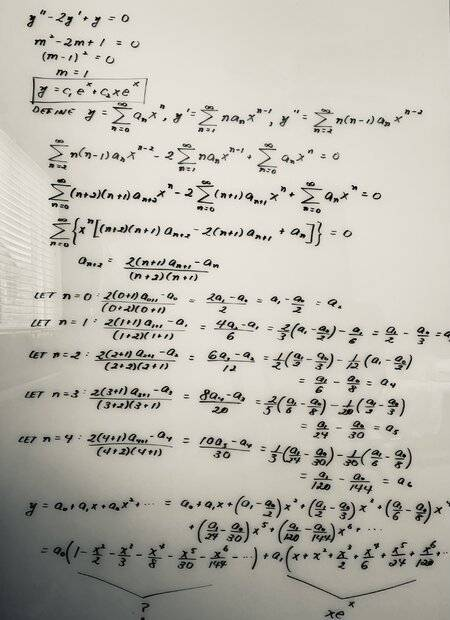# Series Solution for 2nd-Order Homogeneous ODE

• I
• rghurst
In summary, the conversation discusses the solution of a differential equation using two different methods and how they may not give the exact same basis, but should give the same solution for given initial or boundary conditions.

#### rghurst

TL;DR Summary
Can someone please explain why the solution provided by the characteristic equation does not entirely match the series solution? Thanks.Why do you think it doesn't?

Your solution with $a_0 = 1$ and $a_1 = 0$ is a solution with $y(0) = 1$ and $y'(0) = 0$. $e^x$ doesn't satisfy that, but $$e^x - xe^x = 1 + \sum_{n=1}^\infty \left(\frac1{n!} - \frac{1}{(n-1)!}\right)x^n = 1 + \sum_{n=1}^\infty \frac{1 -n}{n!}x^n$$ does. Is that series familiar?

Alternatively, if you solve the recurrence relation for $a_n$ you find that $$a_n = \frac{(a_1 - a_0)n + a_0}{n!},$$ and then it is easy to see that $$\sum_{n=0}^\infty a_nx^n = (a_1 - a_0)xe^x + a_0e^x.$$

Last edited:
Thanks. This makes better sense to me. I am still admittedly having a difficult
time seeing why the series solution would not match exactly that provided by the characteristic polynomial.

You are representing a vector, $y$, with respect to two different bases. The first basis - the obvious one obtained from the characteristic polynomial - is $\{e^x, xe^x\}$ and the components are $c_1$ and $c_2$. The second basis, obtained from the series solution - is $\{e^x, e^x - xe^x\}$ and the components are $a_0$ and $a_1$. These components are related by $$\begin{pmatrix} c_1 \\ c_2 \end{pmatrix} = \begin{pmatrix} 1 & 0 \\ -1 & 1 \end{pmatrix} \begin{pmatrix} a_0 \\ a_1\end{pmatrix}.$$ You should not expect different solution methods to give you exactly the same basis. However, for given initial or boundary conditions you should expect them to give the same solution.

Last edited:
•Mark44
pasmith said:
You are representing a vector, $y$, with respect to two different bases. The first basis - the obvious one obtained from the characteristic polynomial - is $\{e^x, xe^x\}$ and the components are $c_1$ and $c_2$. The second basis, obtained from the series solution - is $\{e^x, e^x - xe^x\}$ and the components are $a_0$ and $a_1$. These components are related by $$\begin{pmatrix} c_1 \\ c_2 \end{pmatrix} = \begin{pmatrix} 1 & 0 \\ -1 & 1 \end{pmatrix} \begin{pmatrix} a_0 \\ a_1\end{pmatrix}.$$ You should not expect different solution methods to give you exactly the same basis. However, for given initial or boundary conditions you should expect them to give the same solution.
pasmith said:
You are representing a vector, $y$, with respect to two different bases. The first basis - the obvious one obtained from the characteristic polynomial - is $\{e^x, xe^x\}$ and the components are $c_1$ and $c_2$. The second basis, obtained from the series solution - is $\{e^x, e^x - xe^x\}$ and the components are $a_0$ and $a_1$. These components are related by $$\begin{pmatrix} c_1 \\ c_2 \end{pmatrix} = \begin{pmatrix} 1 & 0 \\ -1 & 1 \end{pmatrix} \begin{pmatrix} a_0 \\ a_1\end{pmatrix}.$$ You should not expect different solution methods to give you exactly the same basis. However, for given initial or boundary conditions you should expect them to give the same solution.
This makes full sense to me now. Thanks.